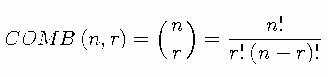# COMB Function

Computes the number of combinations of n elements taken r at a time.

 Category: Combinatorial

## Syntax

COMB(n,r)

### Required Arguments

#### n

is a nonnegative integer that represents the total number of elements from which the sample is chosen.

#### r

is a nonnegative integer that represents the number of chosen elements.

 Restriction r ≤ n

## Details

The mathematical representation of the COMB function is given by the following equation:In the preceding equation, n ≥ 0, r ≥ 0, and nr.
If the expression cannot be computed, a missing value is returned. For moderately large values, it is sometimes not possible to compute the COMB function.

## Example

The following statement produces this result.
SAS Statement
Result
`x=comb(5,1);`
`5`